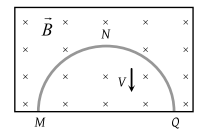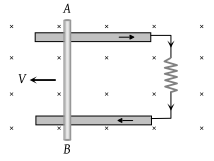A transformer connected to 220 volt line shows an output of 2 A at 11000 volt. The efficiency is 100%. The current drawn from the line is

(1) 100 A

(2) 200 A

(3) 22 A

(4) 11 A

Concept Questions :-

Mutual-inductance
High Yielding Test Series + Question Bank - NEET 2020

Difficulty Level:

A power transformer is used to step up an alternating e.m.f. of 220 V to 11 kV to transmit 4.4 kW of power. If the primary coil has 1000 turns, what is the current rating of the secondary ? Assume 100% efficiency for the transformer

(1) 4 A

(2) 0.4 A

(3) 0.04 A

(4) 0.2 A

Concept Questions :-

Eddy current
High Yielding Test Series + Question Bank - NEET 2020

Difficulty Level:

The primary winding of transformer has 500 turns whereas its secondary has 5000 turns. The primary is connected to an ac supply of 20 V, 50 Hz. The secondary will have an output of

(1) 200 V, 50 Hz

(2) 2 V, 50 Hz

(3) 200 V, 500 Hz

(4) 2 V, 5 Hz

Concept Questions :-

Mutual-inductance
High Yielding Test Series + Question Bank - NEET 2020

Difficulty Level:

A step-down transformer is connected to main supply 200V to operate a 6V, 30W bulb. The current in primary is

(1) 3 A

(2) 1.5 A

(3) 0.3 A

(4) 0.15 A

Concept Questions :-

Mutual-inductance
High Yielding Test Series + Question Bank - NEET 2020

Difficulty Level:

A transformer has 100 turns in the primary coil and carries 8 A current. If input power is one kilowatt, the number of turns required in the secondary coil to have 500V output will be

(1) 100

(2) 200

(3) 400

(4) 300

Concept Questions :-

Mutual-inductance
High Yielding Test Series + Question Bank - NEET 2020

Difficulty Level:

A copper rod of length l is rotated about one end perpendicular to the magnetic field B with constant angular velocity ω. The induced e.m.f. between the two ends is

(1) $\frac{1}{2}B\omega {l}^{2}$

(2) $\frac{3}{4}B\omega {l}^{2}$

(3) $B\omega {l}^{2}$

(4) $2B\omega {l}^{2}$

Concept Questions :-

Motional emf
High Yielding Test Series + Question Bank - NEET 2020

Difficulty Level:

Two conducting circular loops of radii R1 and R2 are placed in the same plane with their centres coinciding. If R1 >> R2, the mutual inductance M between them will be directly proportional to

(1) R1/R2

(2) R2/R1

(3) ${R}_{1}^{2}/{R}_{2}$

(4) ${R}_{2}^{2}/{R}_{1}$

Concept Questions :-

Mutual-inductance
High Yielding Test Series + Question Bank - NEET 2020

Difficulty Level:

A thin semicircular conducting ring of radius R is falling with its plane vertical in a horizontal magnetic induction B. At the position MNQ, the speed of the ring is V and the potential difference developed across the ring is(1) Zero

(2) $B\nu \pi {R}^{2}/2$ and M is at the higher potential

(3) 2RBV and M is at the higher potential

(4) 2RBV and Q is at the higher potential

Concept Questions :-

Motional emf
High Yielding Test Series + Question Bank - NEET 2020

Difficulty Level:

Consider the situation shown in the figure. The wire AB is sliding on the fixed rails with a constant velocity. If the wire AB is replaced by semicircular wire, the magnitude of the induced current will(1) Increase

(2) Remain the same

(3) Decrease

(4) Increase or decrease depending on whether the semicircle bulges towards the resistance or away from it

Concept Questions :-

Motional emf
High Yielding Test Series + Question Bank - NEET 2020

Difficulty Level:

A circular loop of radius R carrying current I lies in the x-y plane with its centre at the origin. The total magnetic flux through the x-y plane is

(1) Directly proportional to I

(2) Directly proportional to R

(3) Directly proportional to R2

(4) Zero

Concept Questions :-

Magnetic flux